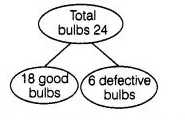# A carton of 24 bulbs contain 6 defective bulbs.

Question:

A carton of 24 bulbs contain 6 defective bulbs. One bulb is drawn at random. What is the probability that the bulb is not defective? If the bulb selected is

defective and it is not replaced and a second bulb is selected at random from the rest, what is the probability that the second bulb is defective?

Solution:

∴Total number of bulbs, n (S) = 24Let $E_{1}=$ Event of selecting not defective bulb $=$ Event of selecting good bulbs

$n\left(E_{1}\right)=18$

$\therefore$ $P\left(E_{1}\right)=\frac{n\left(E_{1}\right)}{n(S)}=\frac{18}{24}=\frac{3}{4}$

Suppose, the selected bulb is defective and not replaced, then total number of bulbs remains in a carton, $n(S)=23$.

In them, 18 are good bulbs and 5 are defective bulbs.

$\therefore P$ (selecting second defective bulb) $=\frac{5}{23}$